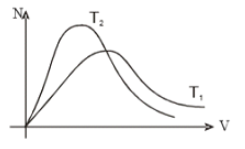${\mathrm{n}}_{1}$ mole of monoatomic gas is mixed with ${\mathrm{n}}_{2}$ mole of diatomic gas such that ${\gamma }_{mix}=1.5$

(1) ${\mathrm{n}}_{1}=2{\mathrm{n}}_{2}$

(2) ${\mathrm{n}}_{2}=2{\mathrm{n}}_{1}$

(3) ${n}_{1}={n}_{2}$

(4) ${n}_{1}=3{n}_{2}$

Concept Questions :-

Specific heat
High Yielding Test Series + Question Bank - NEET 2020

Difficulty Level:

1.

2.

3.

4.  None of these

Concept Questions :-

Specific heat
High Yielding Test Series + Question Bank - NEET 2020

Difficulty Level:

Two chambers containin m1g and m2g of a gas at pressure P1 and P2 respectively are put in contact with each other. If temperature remains constant, the common pressure reached will be

1.

2.

3.

4.

Concept Questions :-

Ideal gas
High Yielding Test Series + Question Bank - NEET 2020

Difficulty Level:

A rocket is propelled by a gas which is initially at a temperature of 4000 K. The temperature of the gas falls to 1000 K as it leaves the exhaust nozzle. The gas which will acquire the largest momentum while leaving the nozzle is:

(1) Hydrogen

(2) Helium

(3) Nitrogen

(4) Argon

Concept Questions :-

Types of velocity
High Yielding Test Series + Question Bank - NEET 2020

Difficulty Level:

The mass of 1 liter of He under a pressure 2 atmosphere and temperature  is:

1.  0.16 g

2.  0.32 g

3.  0.48 g

5.  0.64 g

Concept Questions :-

Ideal gas
High Yielding Test Series + Question Bank - NEET 2020

Difficulty Level:

Maxwell's velocity distribution curve is given for two different temperature. For the given curves-(1) ${T}_{1}>{T}_{2}$

(2) ${T}_{1}<{T}_{2}$

(3) ${T}_{1}\le {T}_{2}$

(4) ${T}_{1}={T}_{2}$

Concept Questions :-

Maxwell distribution curve
High Yielding Test Series + Question Bank - NEET 2020

Difficulty Level:

If P is the pressure of the gas then the KE per unit volume of the gas is:

1.

2.

3.

4.

Concept Questions :-

Kinetic energy of gas
High Yielding Test Series + Question Bank - NEET 2020

Difficulty Level:

The root mean square speed of oxygen molecules (O2) at a certain absolute temperature is v. If the temperature is doubled and oxygen gas dissociates into oxygen atom, the rms speed would be :

1.

2.

3.

4.  $2\sqrt{2}\mathrm{v}$

Concept Questions :-

Types of velocity
High Yielding Test Series + Question Bank - NEET 2020

Difficulty Level:

A gas mixture consist of 2 moles of ${O}_{2}$ and 4 moles of Ar at temperature T. Neglecting all vibrational modes, the total internal energy of the system is:

(a)4RT

(b) 15RT

(c)9RT

(d)11RT

Concept Questions :-

Kinetic energy of gas
High Yielding Test Series + Question Bank - NEET 2020

Difficulty Level:

One mole of an ideal monatomic gas undergoes a process described by the equation $P{V}^{3}=$ constant. The heat capacity of the gas during this process is:

(a) $\frac{3}{2}R$            (b) $\frac{5}{2}R$

(c) $2R$              (d) $R$

Concept Questions :-

Specific heat